# Extra Questions for Maths Circles### Extra Questions for Maths Circles with Answers:

Question: A circle touches the sides of a quadrilateral ABCD at P, Q, R, S respectively. Show that the angles subtended at the centre by a pair of opposite sides are supplementary.

Question: Two circles intersect at two points A and B. AD and AC are diameters to the two circles (in figure). Prove that B lies on the line segment DC.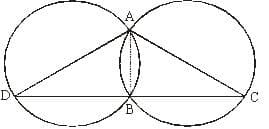Question: Prove equal chords of a circle subtend equal angles at the centre.

Question: A triangle ABC has incentre I and the incircle touches BC, CA at D,E respectively. If BI meets DE in G, show that AG is perpendicular to BG.

Question: ABC and ADC are two right triangles with common hypotenuse AC. Prove that ∠CAD = ∠CBD.

Question: Let P be an interior point of an equilateral triangle ABC such that  Prove that ∠BPC=1500

Question: Two circles intersect at two points B and C. Through B, two line segments ABD and PBQ are drawn to intersect the circles at A, D and P, Q respectively. Prove that ∠ACP = ∠ QCD.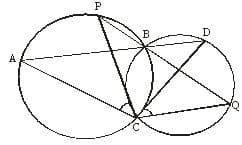Question: If two circles intersect at two points, prove that their centres lie on the perpendicular bisector of the common chord.

Question: In the following figure, AB is a diameter of the circle with centre O, If AC and BD are perpendiculars on a line PQ, and BD meets the circle at E, prove that AC = ED.

Question: A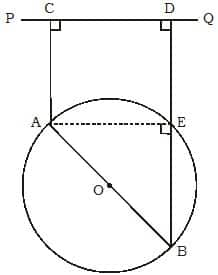Question: If two intersecting chords of a circle make equal angles with the diameter passing through their point of intersection, prove that the chords are equal.

Question: In the given figure, AB is a diameter of the circle C (O, r) and the radius OD is perpendicular to AB. If C is any point on arc DB, find ∠ACD and ∠BAD.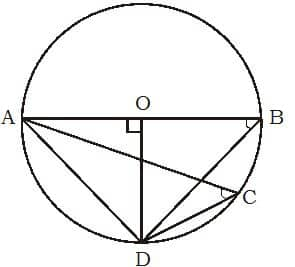Question: Two circles of radii 5 cm and 3 cm intersect at two points and the distance between their centres is 4 cm. Find the length of the common chord.

Question: D is a point on the circumcircle of ABC in which AB = AC such that B and D are on opposite sides of line AC. If CD is produced to a point E such that CE = BD, prove that AD = AE.

Question: Prove that the quadrilateral formed (if possible) by the internal angle bisectors of any quadrilateral is cyclic.

Question: If PAB is a secant to a circle intersecting the circle at A and B and PT is a tangent segment then Prove that PA.PB = PT2.

Question: Prove that the angle subtended by an arc at the centre is double the angle subtended by it at any point on the remaining part of the circle.

Related: wave basics quiz

Question: Let ABC be a triangle, AD the altitude through A and AK the circumdiameter through A. Then prove that ∠DAK = ∠B – ∠C. And also the angular bisector AX of A bisects ∠DAK.

Question: In given figure, ∠ABC = 69o, ∠ACB = 31o, find ∠BDC.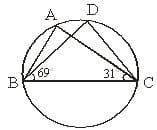Question: If S is the circumcentre of a △ABC, AS meets BC at M meets CA at N and CS meets AB at P, Prove that 1/AM + 1/BN + 1/CP = 2/R, where R is the circum-radius.

Question: Three girls Ruchika, Sonika and Monika are playing a game by standing on a circle of radius 5 m drawn in a park. Ruchika throws a ball to Sonika, Sonika to Monika, Monika to Ruchika. If the distance between Ruchika and Sonika and between Sonika and Monika is 6 m each, what is the distance between Ruchika and Monika?

Question: If the non-parallel sides of a trapezium are equal, prove that it is cyclic.

Question: Find the length of a chord which is at a distance of 8 cm from the centre of a circle of radius 17 cm.

Question: If two equal chords of a circle intersect within the circle, prove that the line joining the point of intersection to the centre makes equal angles with the chords.

Ques. If the radius of a circle is increased to 3 times. Then how many times will its circumference be increased?
(a) 2 times
(b) 1/3 times
(c) 9 times
(d) 3 times

Ans. (d)

#### Yash Bansal

Yash is co-founder of Examsegg. Yash loves to solve questions on reasoning and mathematics. He taught himself how to blend different techniques to get accurate solution in lesser time.